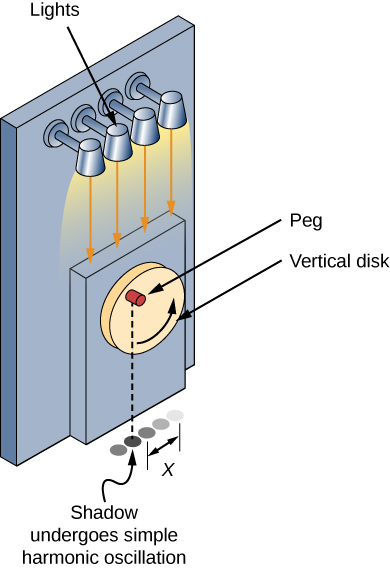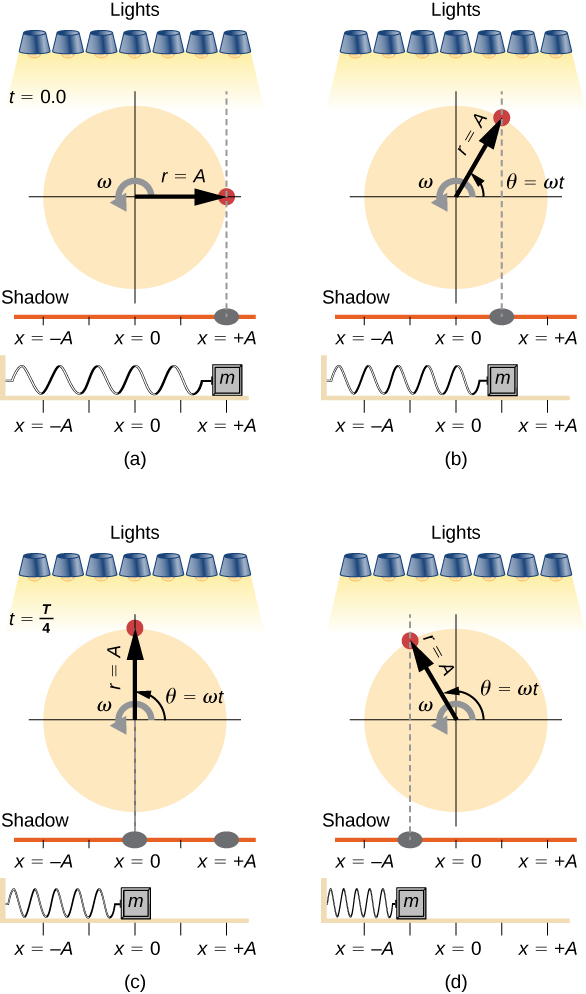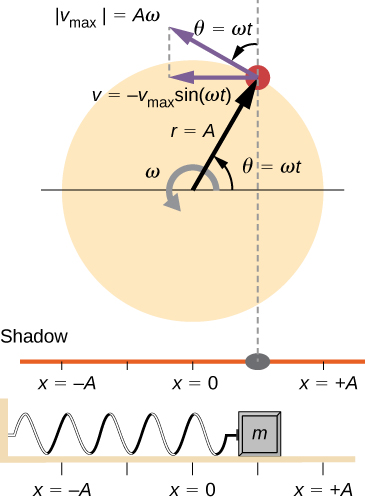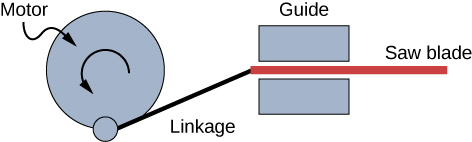## 15.3 Comparing Simple Harmonic Motion and Circular Motion

### Learning Objectives

By the end of this section, you will be able to:

• Describe how the sine and cosine functions relate to the concepts of circular motion
• Describe the connection between simple harmonic motion and circular motion

An easy way to model SHM is by considering uniform circular motion. (Figure) shows one way of using this method. A peg (a cylinder of wood) is attached to a vertical disk, rotating with a constant angular frequency. (Figure) shows a side view of the disk and peg. If a lamp is placed above the disk and peg, the peg produces a shadow. Let the disk have a radius of $r=A$ and define the position of the shadow that coincides with the center line of the disk to be $x=0.00\,m$. As the disk rotates at a constant rate, the shadow oscillates between $x=+A$ and $x=\text{−}A$. Now imagine a block on a spring beneath the floor as shown in (Figure).Figure 15.17 SHM can be modeled as rotational motion by looking at the shadow of a peg on a wheel rotating at a constant angular frequency.Figure 15.18 Light shines down on the disk so that the peg makes a shadow. If the disk rotates at just the right angular frequency, the shadow follows the motion of the block on a spring. If there is no energy dissipated due to nonconservative forces, the block and the shadow will oscillate back and forth in unison. In this figure, four snapshots are taken at four different times. (a) The wheel starts at $\theta ={0}^{o}$ and the shadow of the peg is at $x=+A$, representing the mass at position $x=+A$. (b) As the disk rotates through an angle $\theta =\omega t$, the shadow of the peg is between $x=+A$ and $x=0$. (c) The disk continues to rotate until $\theta ={90}^{0}$, at which the shadow follows the mass to $x=0$. (d) The disk continues to rotate, the shadow follows the position of the mass.

If the disk turns at the proper angular frequency, the shadow follows along with the block. The position of the shadow can be modeled with the equation

$x(t)=A\text{cos}(\omega t).$

Recall that the block attached to the spring does not move at a constant velocity. How often does the wheel have to turn to have the peg’s shadow always on the block? The disk must turn at a constant angular frequency equal to $2\pi$ times the frequency of oscillation $(\omega =2\pi f)$.

(Figure) shows the basic relationship between uniform circular motion and SHM. The peg lies at the tip of the radius, a distance A from the center of the disk. The x-axis is defined by a line drawn parallel to the ground, cutting the disk in half. The y-axis (not shown) is defined by a line perpendicular to the ground, cutting the disk into a left half and a right half. The center of the disk is the point $(x=0,y=0).$ The projection of the position of the peg onto the fixed x-axis gives the position of the shadow, which undergoes SHM analogous to the system of the block and spring. At the time shown in the figure, the projection has position x and moves to the left with velocity v. The tangential velocity of the peg around the circle equals ${\overset{\text{–}}{v}}_{\text{max}}$ of the block on the spring. The x-component of the velocity is equal to the velocity of the block on the spring.Figure 15.19 A peg moving on a circular path with a constant angular velocity $\omega$ is undergoing uniform circular motion. Its projection on the x-axis undergoes SHM. Also shown is the velocity of the peg around the circle, ${v}_{\text{max}}$, and its projection, which is v. Note that these velocities form a similar triangle to the displacement triangle.

We can use (Figure) to analyze the velocity of the shadow as the disk rotates. The peg moves in a circle with a speed of ${v}_{\text{max}}=A\omega$. The shadow moves with a velocity equal to the component of the peg’s velocity that is parallel to the surface where the shadow is being produced:

$v=\text{−}{v}_{\text{max}}\text{sin}(\omega t).$

It follows that the acceleration is

$a=\text{−}{a}_{\text{max}}\text{cos}(\omega t).$

Identify an object that undergoes uniform circular motion. Describe how you could trace the SHM of this object.

### Summary

• A projection of uniform circular motion undergoes simple harmonic oscillation.
• Consider a circle with a radius A, moving at a constant angular speed $\omega$. A point on the edge of the circle moves at a constant tangential speed of ${v}_{\text{max}}=A\omega$. The projection of the radius onto the x-axis is $x(t)=A\text{cos}(\omega t+\varphi )$, where $(\varphi )$ is the phase shift. The x-component of the tangential velocity is $v(t)=\text{−}A\omega \text{sin}(\omega t+\varphi )$.

### Conceptual Questions

Can this analogy of SHM to circular motion be carried out with an object oscillating on a spring vertically hung from the ceiling? Why or why not? If given the choice, would you prefer to use a sine function or a cosine function to model the motion?

If the maximum speed of the mass attached to a spring, oscillating on a frictionless table, was increased, what characteristics of the rotating disk would need to be changed?

### Problems

The motion of a mass on a spring hung vertically, where the mass oscillates up and down, can also be modeled using the rotating disk. Instead of the lights being placed horizontally along the top and pointing down, place the lights vertically and have the lights shine on the side of the rotating disk. A shadow will be produced on a nearby wall, and will move up and down. Write the equations of motion for the shadow taking the position at $t=0.0\,\text{s}$ to be $y=0.0\,\text{m}$ with the mass moving in the positive y-direction.

(a) A novelty clock has a 0.0100-kg-mass object bouncing on a spring that has a force constant of 1.25 N/m. What is the maximum velocity of the object if the object bounces 3.00 cm above and below its equilibrium position? (b) How many joules of kinetic energy does the object have at its maximum velocity?

Reciprocating motion uses the rotation of a motor to produce linear motion up and down or back and forth. This is how a reciprocating saw operates, as shown below.If the motor rotates at 60 Hz and has a radius of 3.0 cm, estimate the maximum speed of the saw blade as it moves up and down. This design is known as a scotch yoke.

A student stands on the edge of a merry-go-round which rotates five times a minute and has a radius of two meters one evening as the sun is setting. The student produces a shadow on the nearby building. (a) Write an equation for the position of the shadow. (b) Write an equation for the velocity of the shadow.